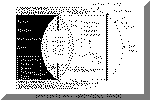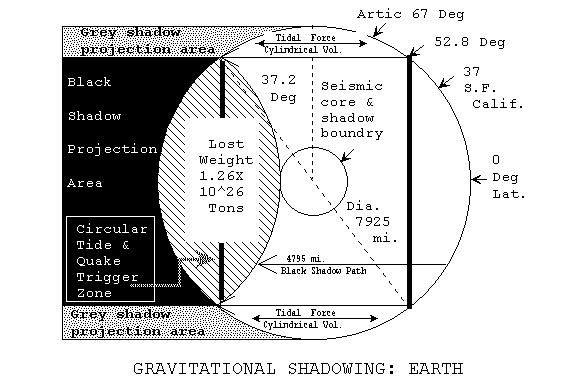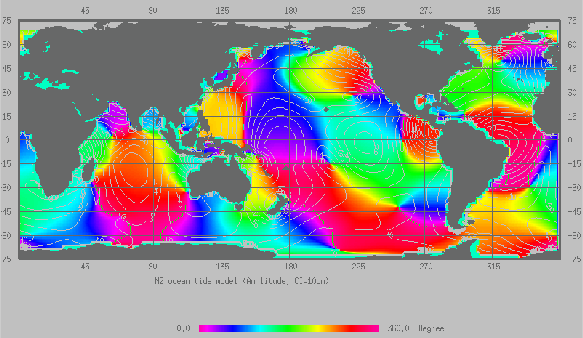Gravity Concepts, Sec. 30, Rev. May 12, 2015    ///

Home          < Gravity Concepts ># Gravity Shielding

Site Page List         Site Images        < Radiant Press

### TOTAL SHIELDING PATH LENGTH

The position of the Earth and Venus on the densare curve, FIGURE 1, suggests that their projected shadows may consist of black centers with a gray circumference. The overall average value is made up of the black portion and the gray area. The black portion would have the limited densare shadowing of 1.52X10exp.14 tons mass per square mile. The graduated gray area would have an average value dependent on the width of the gray area.

Since Earth has an average densare of 1.335 X10 exp 14 tons per square mile, and the space maximum is 1.52_X10_exp_14 it is possible to calculate the area of the black shadow shielding for material of Earth's density. The black shadow path length for a given material is determined by the depth of matter the radiation must penetrate before its ability to produce gravitational force is expended. The black shadow path for Earth, LBS, was calculated to be 7715 km,  (4,795 miles), assuming the Earth has a homogeneous density.

The calculations used to determine this path length are given in Appendix 1. FIGURE 2 presents the geometric relations between the calculated black shadow area, path length and the Earth's geometry. If one calculates the black shadow path for Venus, a different path length must be expected, unless Venus consists of the same material with the same compaction and density as that of Earth.

< Prev Pg   <Contents    /\Top Pg   Bottom\/Note:  Inner core boundary 1330 km, ( 827 mile) radius per model.                  Seismic core boundary 1383 km,  (860 mile) radius measured.

Figure  2

Earthquake activity and global tidal action may be more pronounced along the circular boundary of the black shadow area. The area and volume within the black shadow is subjected to a constant gravitational interaction with the Sun and Moon.### TOTAL SHIELDING PATH LENGTH cont.

The area and volume in the gray shadow area is subjected to a tangential gravitational interaction toward the Moon. This model mechanically predicts that this moving boundary will be coincident with earthquake activity, the Earths tidal profile and tidal discontinuances.  This global tidal range map provides a course view of the maximum tidal variations.

If the Earth were a smooth sphere covered with a layer of water, this shadowing model mechanically predicts the tidal profile for the side facing the moon would be a large ring structure, inside the black shadow area, and have a depressed center.  If the Earth were not spinning the center of the depression would be directly under the Moon.  As viewed from the Moon the tidal structure would appear as a large impact crater. The depressed center directly under the Moon results from prime radiation shielding by the Moon. The Earth's surface gravity is normally at 1 g due to the shielding below and a full degree of radiation from above. This gives the maximum radiation flow unbalance which is the cause of the gravitational force. This shielding by the Moon reduces the Earth's normal surface gravity for that area due to the reduced radiation from the zenith. A reduced surface gravity will result in reducing the ocean level for that area. The build up of the volume in the ring structure would result from the tangential force toward the Moon on the ocean volume that lies outside the black shadow projection area.

A tidal bulge opposite the Moon side would be predicted by this model for the area inside the black shadow boundary. This would be due to the common centrifugal force resulting from the Earth and Moon orbiting around the common barycenter.  On this far side the liquid in the gray shadow projection boundary would also be subject to tangential force toward the Moon.

The history of quake activity data in relation to the known Sun and Moon positions should accurately plot the black shadow boundary as related to the solar system body. It is to be noted that a circular black shadow boundary exists on the surface facing the opposing solar system body and also on the opposite side of the Earth. Each of these circular boundaries should be a potential earthquake trigger zone. The polar areas are not exposed to these moving boundaries and are not subjected to the frequent earthquake triggers.  The polar areas would still have crustal stress and earthquakes from plate movement but would not be exposed to the frequent trigger forces.

### TOTAL SHIELDING PATH LENGTH cont.

Figure 2B presents a graph of 9036 earthquakes greater then level 5 for the period between July 1994 to December 1996. It is scaled in the number of quakes per two degrees Latitude for the 30 month period.### Fig. 2B     Absence of Quakes at Poles

Seismic measurements within the earth indicate a refraction of sound waves at the boundary of what is normally called the inner core. This refraction is conventionally attributed to a core that is thought to be of a higher density than the surrounding material. It is of interest to note that this refraction boundary forms a surface that has essentially the same radius as the boundary between the totally shielded matter and the normal unshielded matter. The black shadow path calculations put this surface radius at 827 miles. The inner core measurements put the radius at 860 miles. These measurements show that the inner core boundary position matches the calculated black shadow path length within a one half percent error. Therefore it is possible that the seismic measurements are actually indicating the boundary between the shielded and unshielded matter.

From the  Calculations: Black Shadow Path Length A3-A7 the following table of EARTH SHADOW CHARACTERISTICS were determined, TABLE 3. The values listed are expected to vary somewhat from the actual, since an average homogeneous density was assumed. In this list mass shielding refers to the matter being shielded from the effects of gravitational forces between planetary bodies. Inertial mass shielding is treated in a later section of this paper.

< Prev Pg   < Contents    /\Top Pg   Bottom\/

 Area of Projected            Black Shadow ABS = 0.634 x Proj. Area Earth, = 3.133 ep7 sq.mi.* Area of Gray Shadow, AGS = 0.366 x Proj. Area Earth, = 1.8 ep7 sq. mi Length of Black Shadow LBS = O.604 Dia. Earth = 4795 miles Volume Shielded by  Black Shadow V Lost = 0.16 x Vol. Earth = 4.17 ep10 cu. mi. Actual Specific Gravity of Earth Sga = 6.55 Norm Apparent Sp. Grav. of Earth Sgn = 5.5 Mass Actual of Total Matter M act = 7.84 ep21 tons Mass (weight) Projected Earth Me  = 83.9% M act, = 6.58 ep21 tons Mass Shielded, M Lost M Lost = 16.1% M act, = 1.26 ep21 tons Note *  ep = 1 x 10^